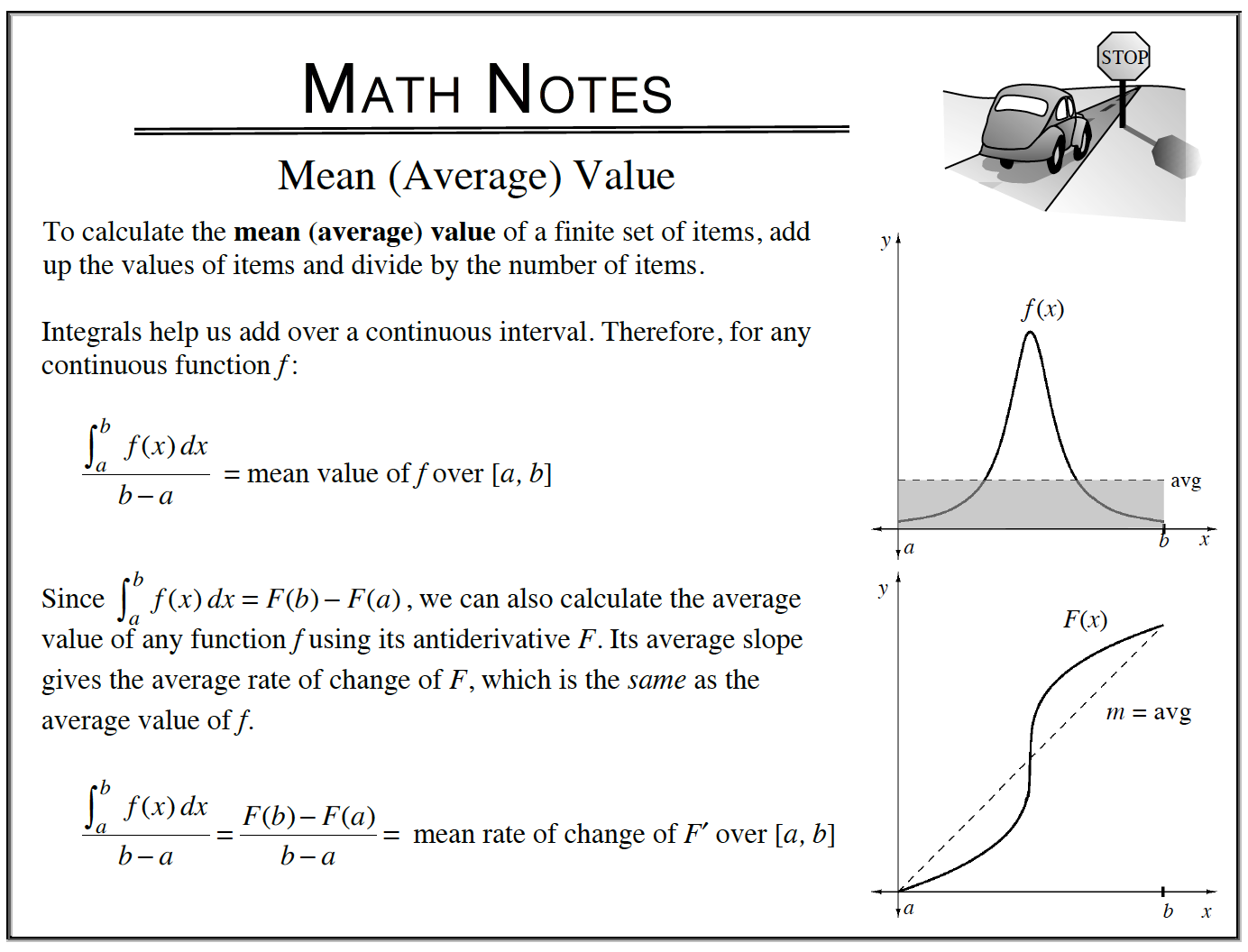Home > APCALC > Chapter 7 > Lesson 7.1.4 > Problem7-42

7-42.

Kimberly and Varag are in a bicycle race. Homework Help ✎

For parts (a) and (b), each is a different application of Mean Value. Pay attention to the given information and read carefully.
See Math Notes below.

1. If Kimberly’s velocity in miles per hour during the race is $v(t) = 2.3^t\sin( \frac { t } { 2 } )$, calculate her average velocity during $0 ≤ t ≤ 6$. Describe your method.

Average Velocity = 16.102 mph

2. If Varag’s distance from the starting line during the race is $s(t) = 20t + 2\sin(t)$, calculate his average velocity during $0 ≤ t ≤ 6$. Describe your method.

Average Velocity $= 19.907$ mph

3. When is each bicyclist traveling at his/her average velocity?

This is an application of Mean Value Theorem, which states that a function (such as a velocity function) must equal its average value at least once on a closed interval (that is, if the function is continuous and differentiable).
See Math Notes below.